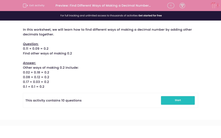# Find Different Ways of Making a Decimal Number by Addition

In this worksheet, students find different ways of making a decimal number by addition of other decimals.This content is premium and exclusive to EdPlace subscribers.Key stage:  KS 2

Curriculum topic:   Maths and Numerical Reasoning

Curriculum subtopic:   Decimals

Difficulty level:#### Worksheet Overview

In this worksheet, we will learn how to find different ways of making a decimal number by adding other decimals together.

Question:

0.11 + 0.09 = 0.2
Find other ways of making 0.2

Other ways of making 0.2 include:

0.02 + 0.18 = 0.2
0.08 + 0.12 = 0.2
0.17 + 0.03 = 0.2
0.1 + 0.1 = 0.2

### What is EdPlace?

We're your National Curriculum aligned online education content provider helping each child succeed in English, maths and science from year 1 to GCSE. With an EdPlace account you’ll be able to track and measure progress, helping each child achieve their best. We build confidence and attainment by personalising each child’s learning at a level that suits them.

Get started